# 正文

``````#!python

import torch
from torch import nn, optim
import numpy as np
import matplotlib.pyplot as plt
from torch.nn.parameter import Parameter

x_train = np.array([[3.3], [4.4], [5.5], [6.71], [6.93], [4.168],
[9.779], [6.182], [7.59], [2.167], [7.042],
[10.791], [5.313], [7.997], [3.1]], dtype=np.float32)

y_train = np.array([[1.7], [2.76], [2.09], [3.19], [1.694], [1.573],
[3.366], [2.596], [2.53], [1.221], [2.827],
[3.465], [1.65], [2.904], [1.3]], dtype=np.float32)

x_train = torch.from_numpy(x_train)

y_train = torch.from_numpy(y_train)

class LinearRegression(nn.Module):
def __init__(self):
super().__init__()
self.linear = nn.Linear(1, 1)

def forward(self, x):
out = self.linear(x)
return out

model = LinearRegression()
criterion = nn.MSELoss()
optimizer = optim.SGD(model.parameters(), lr=1e-2)

num_epochs = 10000

inputs = Variable(x_train)
target = Variable(y_train)
for epoch in range(num_epochs):
out = model(inputs)
loss = criterion(out, target)
loss.backward()
optimizer.step()

if (epoch+1) % 20 == 0:
print("Epoch[%d/%d], loss: {:%.6f}"%(epoch+1, num_epochs, loss.data))

model.eval()
predict = model(x_train)
predict = predict.data.numpy()
plt.plot(x_train.numpy(), y_train.numpy(), 'ro', label='Original data')
plt.plot(x_train.numpy(), predict, label='Fitting Line')
plt.legend()
plt.show()
``````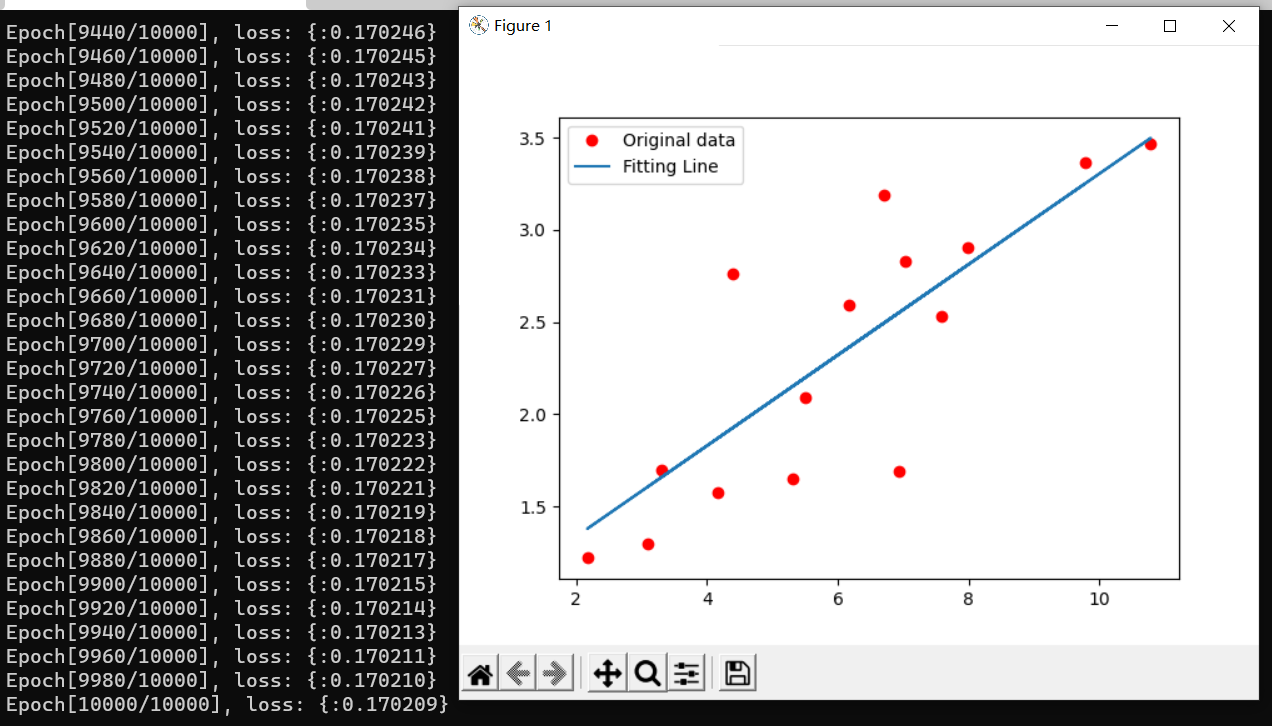## import

``````#!python

import torch
from torch import nn, optim
import numpy as np
import matplotlib.pyplot as plt``````

## 数据

``````x_train = np.array([[3.3], [4.4], [5.5], [6.71], [6.93], [4.168],
[9.779], [6.182], [7.59], [2.167], [7.042],
[10.791], [5.313], [7.997], [3.1]], dtype=np.float32)

y_train = np.array([[1.7], [2.76], [2.09], [3.19], [1.694], [1.573],
[3.366], [2.596], [2.53], [1.221], [2.827],
[3.465], [1.65], [2.904], [1.3]], dtype=np.float32)

x_train = torch.from_numpy(x_train)

y_train = torch.from_numpy(y_train)``````

``````\$ ./lr.py
tensor([[ 3.3000],
[ 4.4000],
[ 5.5000],
[ 6.7100],
[ 6.9300],
[ 4.1680],
[ 9.7790],
[ 6.1820],
[ 7.5900],
[ 2.1670],
[ 7.0420],
[10.7910],
[ 5.3130],
[ 7.9970],
[ 3.1000]])``````

（张量，就当成n维数组吧？一种作为神经网络输入输出的特殊数组？一维张量是向量，二维张量是矩阵，三维是个体？）
（其实也可以用python自带的array创建张量的，这里用了numpy我猜是信仰问题）

``````\$ ./lr.py
tensor([[ 3.3000,  1.0000],
[ 4.4000,  1.0000],
[ 5.5000,  1.0000],
[ 6.7100,  1.0000],
[ 6.9300,  1.0000],
[ 4.1680,  1.0000],
[ 9.7790,  1.0000],
[ 6.1820,  1.0000],
[ 7.5900,  1.0000],
[ 2.1670,  1.0000],
[ 7.0420,  1.0000],
[10.7910,  1.0000],
[ 5.3130,  1.0000],
[ 7.9970,  1.0000],
[ 3.1000,  1.0000]])``````

`np.array([[3.3],....[x.x]], dtype= ...)`

（当然，这只是最简单的理解方式）

``````x_train = np.array([3.3, 4.4, 5.5, 6.71, 6.93, 4.168,
9.779, 6.182, 7.59, 2.167, 7.042,
10.791, 5.313, 7.997, 3.1], dtype=np.float32)``````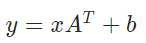`RuntimeError: mat1 and mat2 shapes cannot be multiplied (1x15 and 1x1)`

## 定义网络1 （还是应该叫模型？模块？）

``````class LinearRegression(nn.Module):
def __init__(self):
super().__init__()
self.linear = nn.Linear(1, 1)

def forward(self, x):
out = self.linear(x)
return out``````

``````foo = LinearRegression()
#创建对象，py不需要new，因此总是屑屑的和方法搞混

foo(inputs)
#嗯？这是对象还是方法？
#这是py的特性，只需要在对象中定义def __call__ (self, .....)
#就可以把一个对象像方法一样调用了，是不是很离谱``````

``````def __init__(self):
super().__init__()
self.linear = nn.Linear(1, 1)``````

`super().__init__()`调用父类的构造函数，不看了，又懒又菜

`self.linear = nn.Linear(1, 1)`，这才是关键，或者可以说，是这个网络的实际内容（因为没别的东西了）

`nn.Linear`是一个封装好的，专门用于线性回归的`nn.Module`（没错，我们这个类继承的也是`nn.Module`，这是一个套娃行为），构造参数(1,1)代表，一维输入，一维输出（标准的 y = kx + b），其实它还可以有第三个布尔型参数，代表是否要有b，默认是True，如果指定为False就是强制过原点了。。。

``````model = LinearRegression()
............
....训练....
............
print(model.linear.weight)#这是k
print(model.linear.bias)#这是b``````

``````Parameter containing:
Parameter containing:

## 变量

Variable，是Pytorch自动求导机制和反向传播机制的关键一环

### 额外参数和方法有啥？

• 布尔型 `requires_grad`
• 梯度 （大概是浮点型） `grad`
• 方法的引用 `grad_fn`
• 反向传播方法 `backward()`

### 它是如何工作的？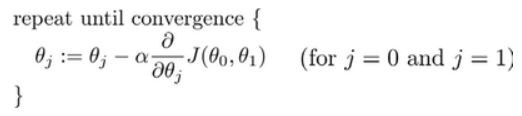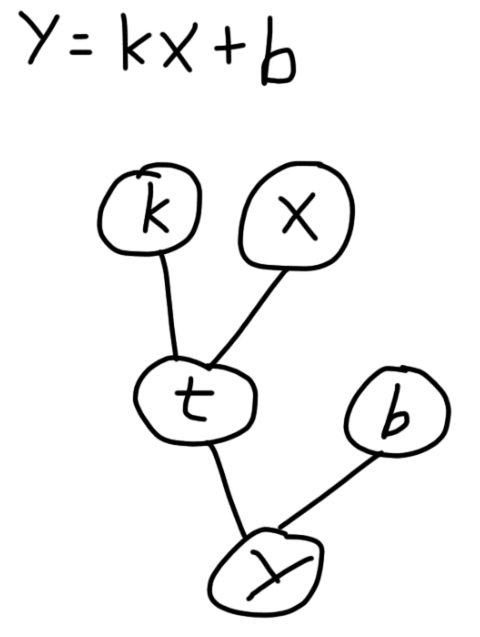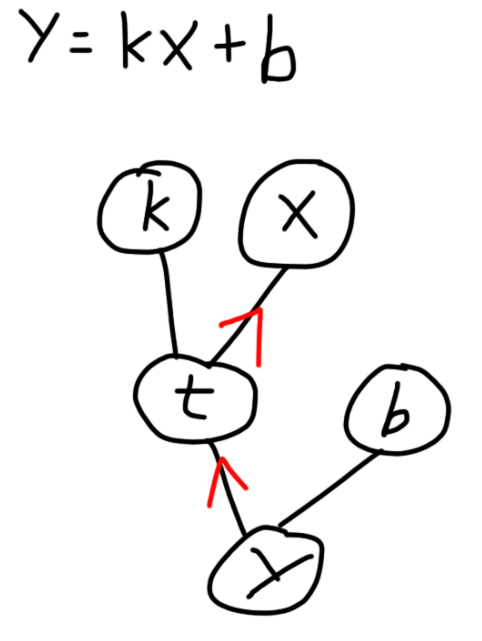``````#!python

import torch

x = Variable(torch.tensor(3.0))
k = Variable(torch.tensor(2.0), requires_grad = True)
b = Variable(torch.tensor(4.0))
y = k ** x + b

y.backward()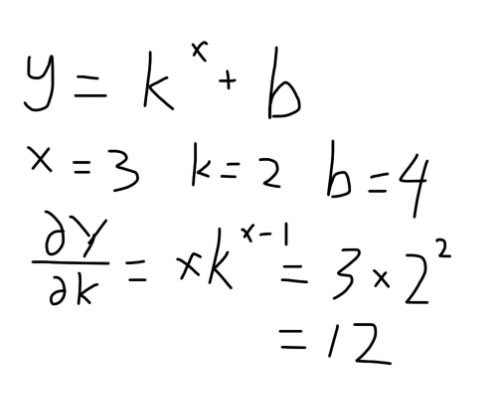``````\$ ./test.py
tensor(12.)``````

## 定义网络2

``````class LinearRegression(nn.Module):
def __init__(self):
super().__init__()
self.linear = nn.Linear(1, 1)

def forward(self, x):
out = self.linear(x)
return out``````

``````def forward(self, x):
out = self.linear(x)
return out``````

“对于一个神经网络模型来说，确定这个模型有哪些层以及这些层之间的数据如何流动，那这个模型就确定了。所以模型的__init__方法声明模型有哪些类，模型的forward方法规定数据如何在层之间流动。”

``````class LinearRegression():
def __init__(self):
super().__init__()
self.linear = nn.Linear(1, 1)

def forward(self, x):
out = self.linear(x)
return out``````

emmm，我觉得直接拿来用是没有任何问题的，反向传播和求梯度也不会产生什么问题，问题只会发生在优化器的使用上 （其实是在盲猜），下面再说

## 损失函数和优化器

``````model = LinearRegression()
criterion = nn.MSELoss()
optimizer = optim.SGD(model.parameters(), lr=1e-2)``````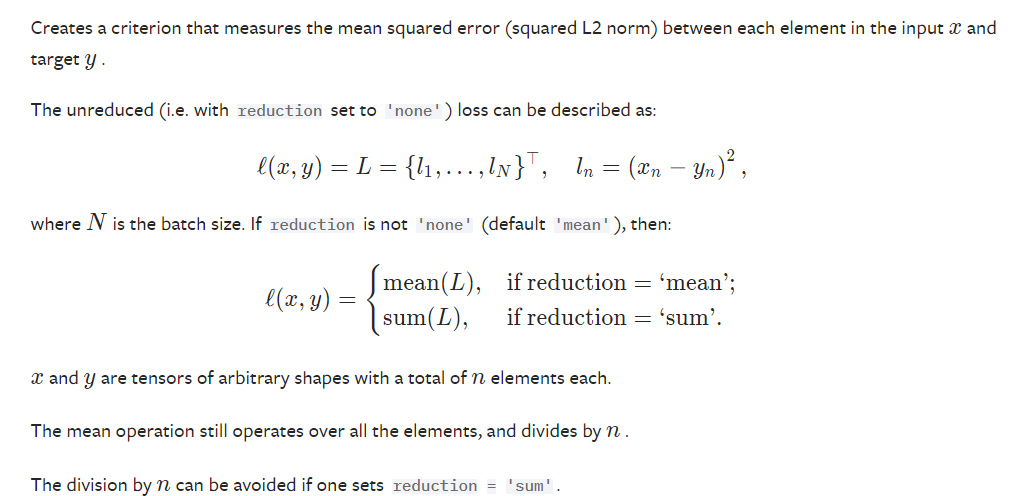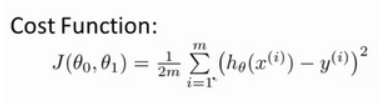`optimizer = optim.SGD(model.parameters(), lr=1e-2)`呢？`self.linear = nn.Linear(1, 1)`

Python为类提供了别致的魔法方法`__setattr__()`，能够轻松的拦截这种给对象加属性的操作，而`nn.Module`正是继承了这一接口，将赋值作为属性的子网络，通通放入了一个子网络列表中，参数就能随便遍历啦

Pytorch提供了`Parameter(tensor)`这个类，能够将一个张量转为一个“网络参数”，它和变量其实并没有什么区别（不过它的`requires_grad`默认就为True），而Parameter的特点就在于，我们把它当作属性赋值给一个网络时，就会像上面的子网络一样，被魔法方法拦截，并增加到参数列表中。

## 开始训练

``````num_epochs = 10000

inputs = Variable(x_train)
target = Variable(y_train)
for epoch in range(num_epochs):
out = model(inputs)
loss = criterion(out, target)
loss.backward()
optimizer.step()

if (epoch+1) % 20 == 0:
print("Epoch[%d/%d], loss: {:%.6f}"%(epoch+1, num_epochs, loss.data))``````

`num_epochs = 10000`，定义了要训练多少次
`inputs = Variable(x_train)``target = Variable(y_train)`则是把输入和输出由单纯的张量转为了变量，不过我试了一下，不转也是没有任何问题的。张量和变量并没有进行什么明显的区分，普通张量也具有`grad``requires_grad`之类的属性，也许变量存在的意义仅限于作为求梯度的对象吧.....嗯，不影响大局，以后有机会再研究吧

`out = model(inputs)`，将数据作为矩阵输入到网络中，一行是一组数据，得到的out是输出，一行为一组输出
`loss = criterion(out, target)`，使用上面定义的代价函数对象，将包含网络算出的结果和正确结果的矩阵传入，一行为一组数据，得到一个0维张量（变量）作为输出（0维张量说白了就是一个数嘛）

`optimizer.zero_grad()`

`loss.backward()`，上面所说的反向传播
`optimizer.step()`，根据上面反向传播，传给参数的梯度，按照优化器的规则（此处就是梯度下降），调整参数内容，改进模型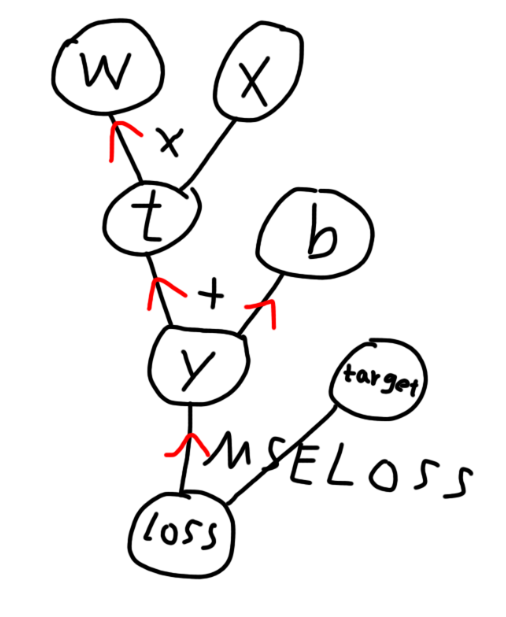## 收尾工作

``````if (epoch+1) % 20 == 0:
print("Epoch[%d/%d], loss: {:%.6f}"%(epoch+1, num_epochs, loss.data))``````

`model.eval()`

``````predict = model(x_train)
predict = predict.data.numpy()
plt.plot(x_train.numpy(), y_train.numpy(), 'ro', label='Original data')
plt.plot(x_train.numpy(), predict, label='Fitting Line')
plt.legend()
plt.show()``````